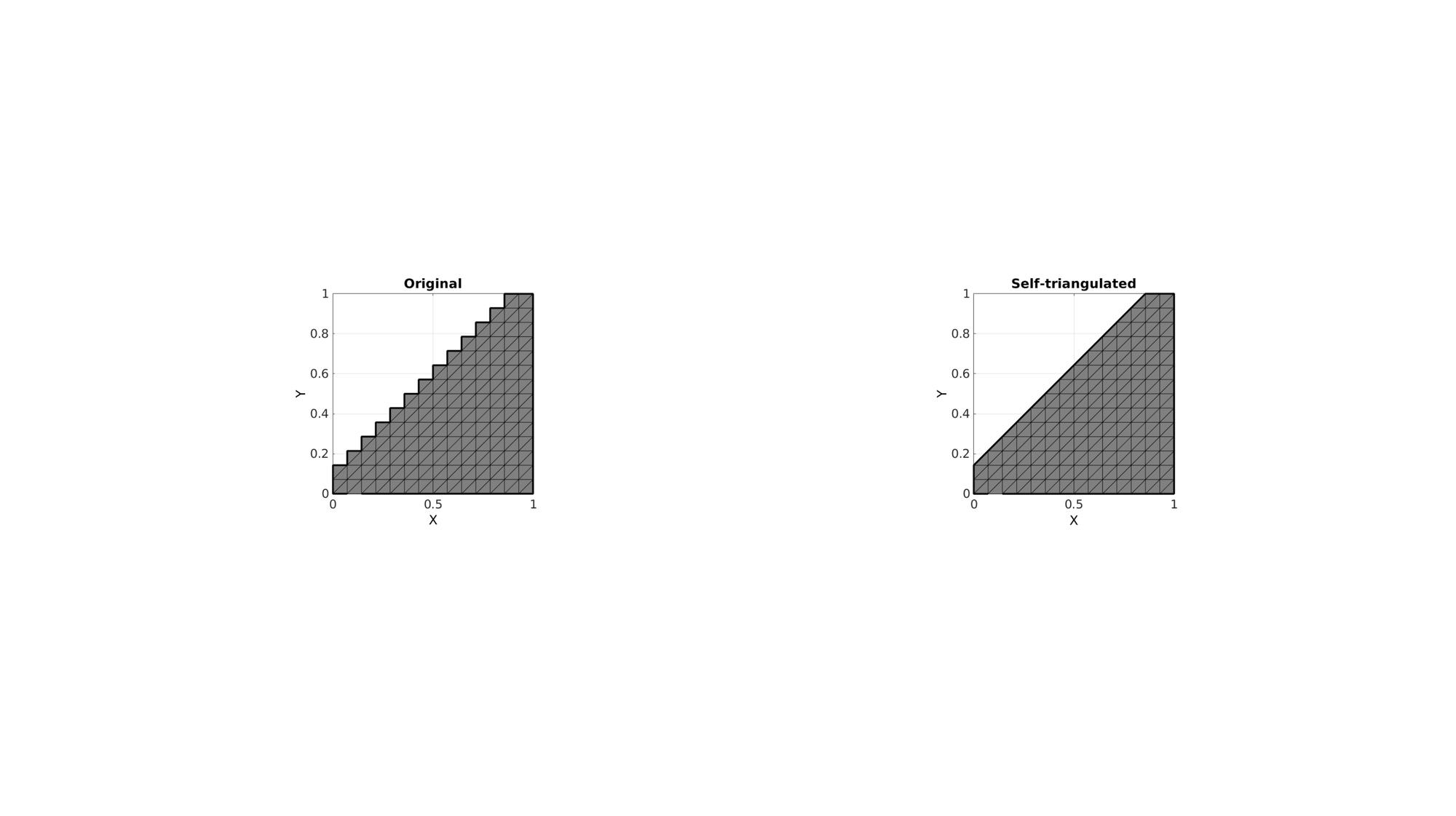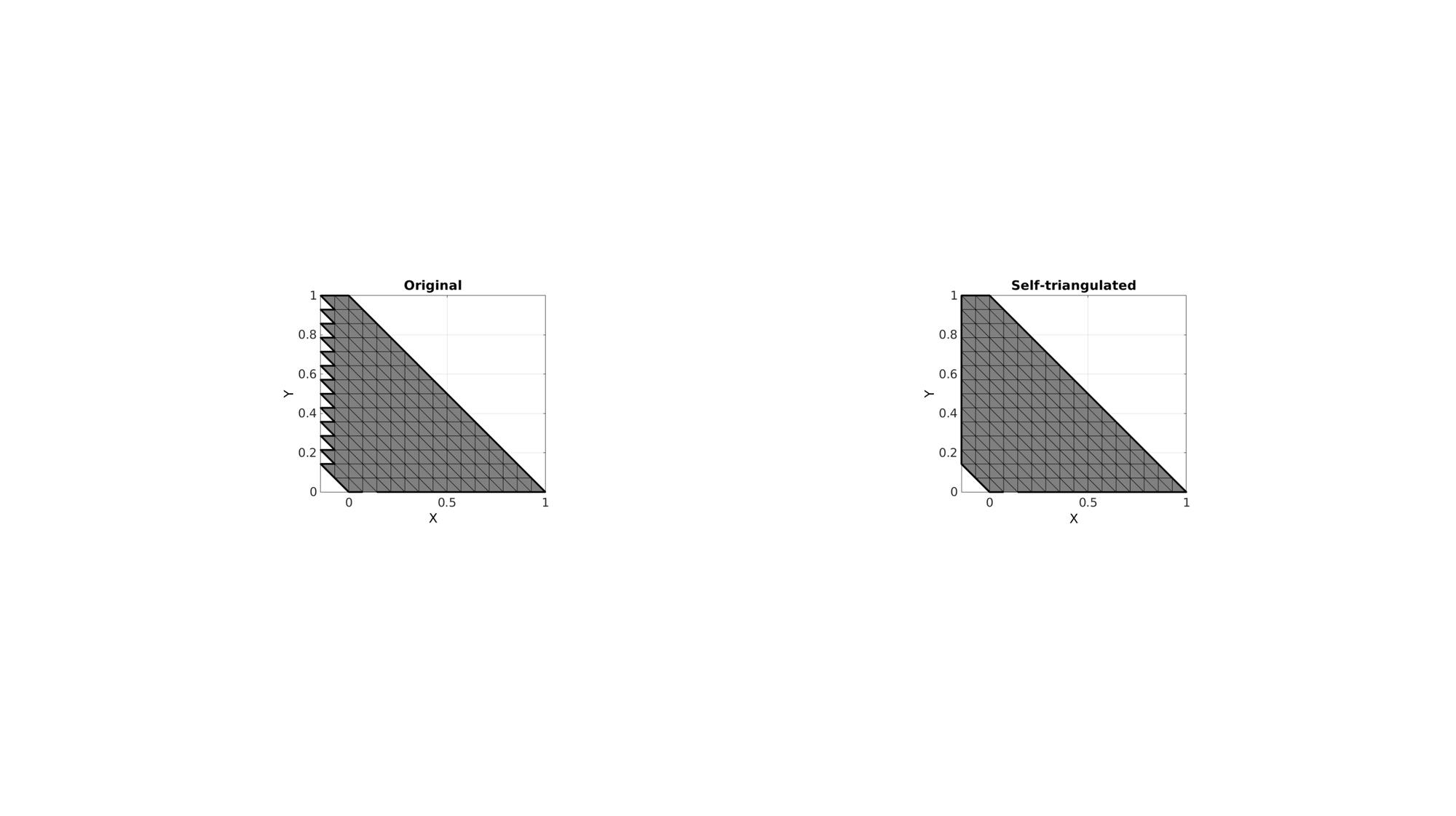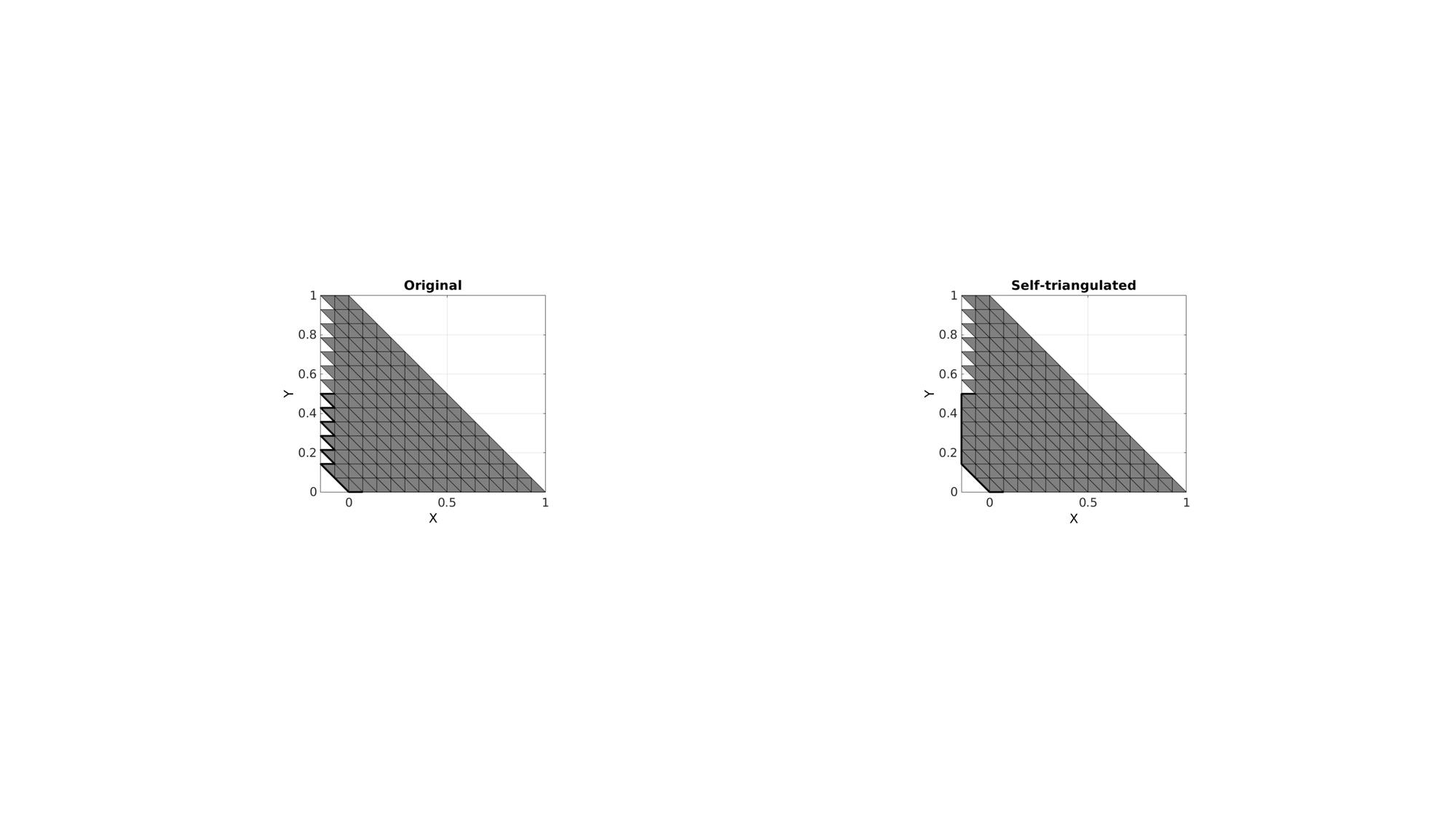# triSurfSelfTriangulateBoundary

Below is a demonstration of the features of the triSurfSelfTriangulateBoundary function

## Contents

```clear; close all; clc;
```

## Syntax

[F1,V1,ind1]=triSurfSelfTriangulateBoundary(F1,V1,ind1,angleThreshold,isClosedLoop);

## Description

The triSurfSelfTriangulateBoundary function

## Example 1: Self-triangulate the boundary of a surface by creating triangles at sharp boundary segments

Create test data set

```w=1;
[X,Y]=ndgrid(linspace(0,w,15));
Z=ones(size(X));
C=tril(Z);
C=vertexToFaceMeasure(F,C)>0;

logicKeep=C>0;
F=F(logicKeep,:);
C=C(logicKeep,:);
[F,V]=patchCleanUnused(F,V);

F=[F(:,[1 2 3]);F(:,[3 4 1])]; %Triangles
```

Get boundary curve

```Eb=patchBoundary(F,V);
indBoundaryCurve=edgeListToCurve(Eb);
indBoundaryCurve=indBoundaryCurve(1:end-1)'; %Start=End for closed curve so remove double entry
```

Self triangulate

```angleThreshold=(100/180)*pi;
isClosedPath=1;
[F_new,V_new,indBoundaryCurve_new]=triSurfSelfTriangulateBoundary(F,V,indBoundaryCurve,angleThreshold,isClosedPath);
```
```cFigure;
subplot(1,2,1); hold on;
title('Original');
gpatch(F,V,'kw');
plotV(V(indBoundaryCurve,:),'k-','LineWidth',3);
axisGeom; view(2);

subplot(1,2,2); hold on;
title('Self-triangulated');
gpatch(F_new,V_new,'kw');
plotV(V_new(indBoundaryCurve_new,:),'k-','LineWidth',3);
axisGeom; view(2);

drawnow;
```## Example 2: Altered shape

```V(:,1)=V(:,1)-V(:,2);
```

Calculate mesh path angles

```[F_new,V_new,indBoundaryCurve_new]=triSurfSelfTriangulateBoundary(F,V,indBoundaryCurve,angleThreshold,isClosedPath);
```
```cFigure;
subplot(1,2,1); hold on;
title('Original');
gpatch(F,V,'kw');
plotV(V(indBoundaryCurve,:),'k-','LineWidth',3);
axisGeom; view(2);

subplot(1,2,2); hold on;
title('Self-triangulated');
gpatch(F_new,V_new,'kw');
plotV(V_new(indBoundaryCurve_new,:),'k-','LineWidth',3);
axisGeom; view(2);

drawnow;
```## Example 3: Self-triangulate a part of boundary surface

Create path segment

```indBoundaryCurve=indBoundaryCurve(1:15);
```

Calculate mesh path angles

```isClosedPath=0;
[F_new,V_new,indBoundaryCurve_new]=triSurfSelfTriangulateBoundary(F,V,indBoundaryCurve,angleThreshold,isClosedPath);
```
```cFigure;
subplot(1,2,1); hold on;
title('Original');
gpatch(F,V,'kw');
plotV(V(indBoundaryCurve,:),'k-','LineWidth',3);
axisGeom; view(2);

subplot(1,2,2); hold on;
title('Self-triangulated');
gpatch(F_new,V_new,'kw');
plotV(V_new(indBoundaryCurve_new,:),'k-','LineWidth',3);
axisGeom; view(2);

drawnow;
```GIBBON www.gibboncode.org

Kevin Mattheus Moerman, [email protected]

GIBBON footer text

GIBBON: The Geometry and Image-based Bioengineering add-On. A toolbox for image segmentation, image-based modeling, meshing, and finite element analysis.

Copyright (C) 2019 Kevin Mattheus Moerman

This program is free software: you can redistribute it and/or modify it under the terms of the GNU General Public License as published by the Free Software Foundation, either version 3 of the License, or (at your option) any later version.

This program is distributed in the hope that it will be useful, but WITHOUT ANY WARRANTY; without even the implied warranty of MERCHANTABILITY or FITNESS FOR A PARTICULAR PURPOSE. See the GNU General Public License for more details.

You should have received a copy of the GNU General Public License along with this program. If not, see http://www.gnu.org/licenses/.